Ex 11.4

Chapter 11 Class 11 Conic Sections (Term 2)
Serial order wise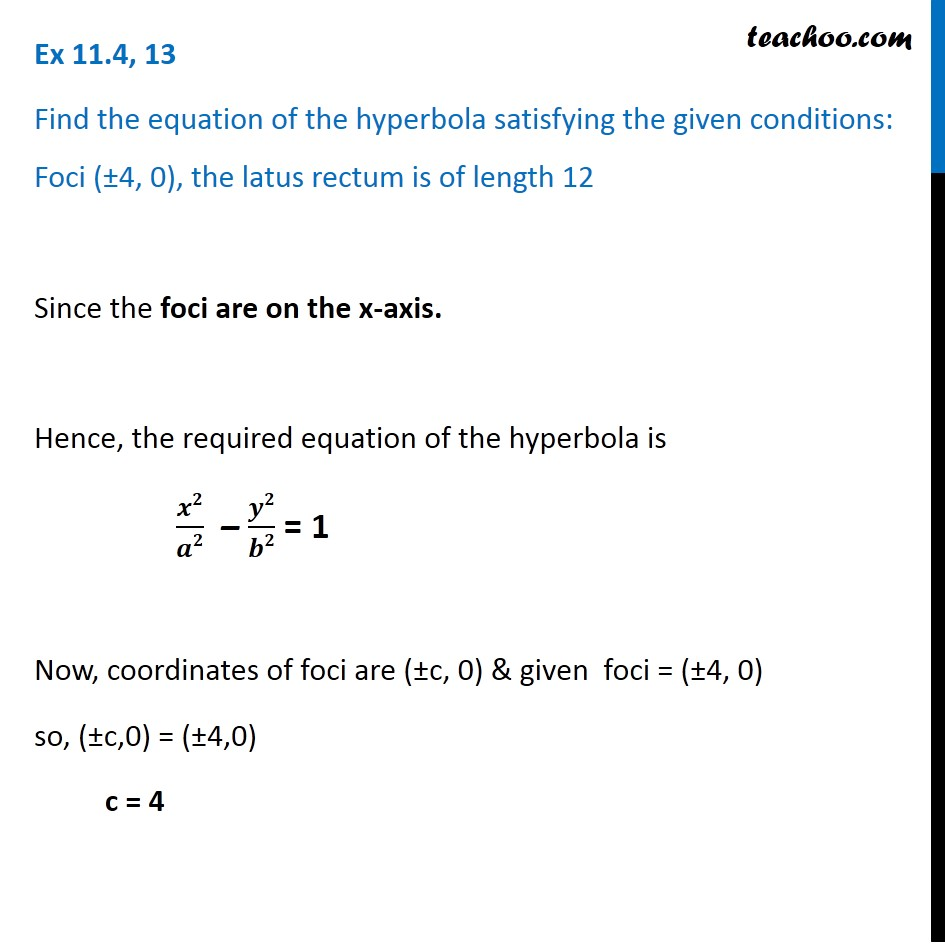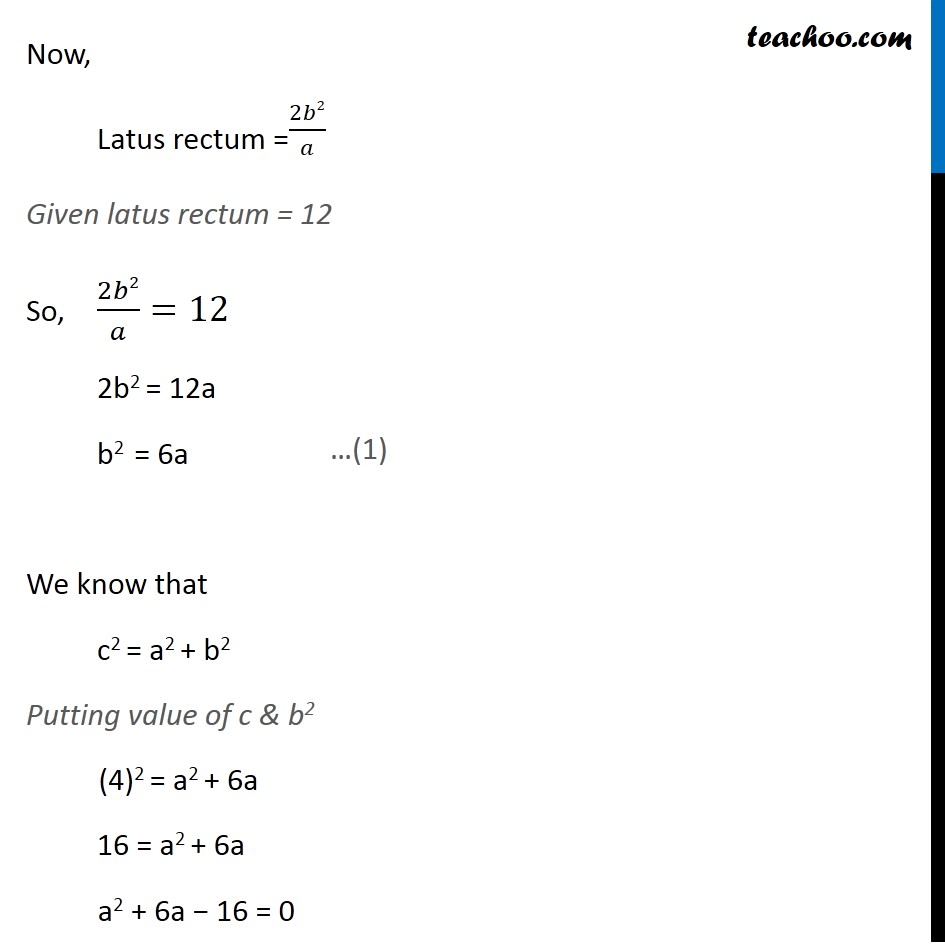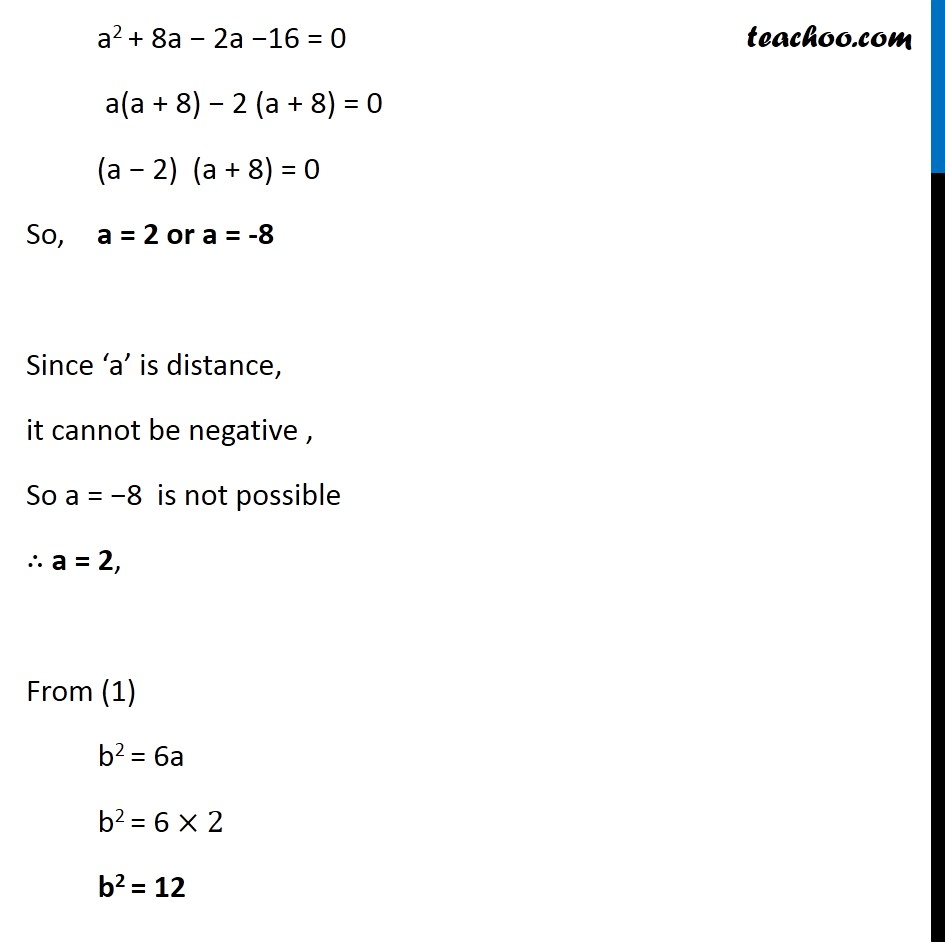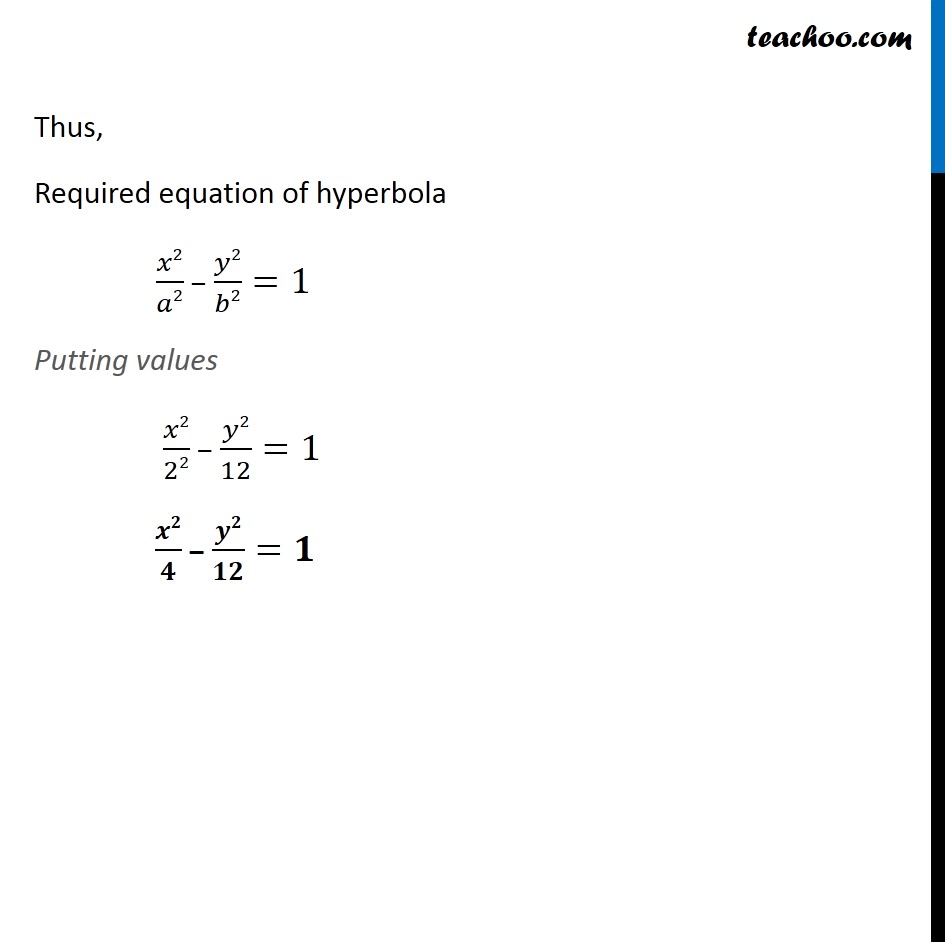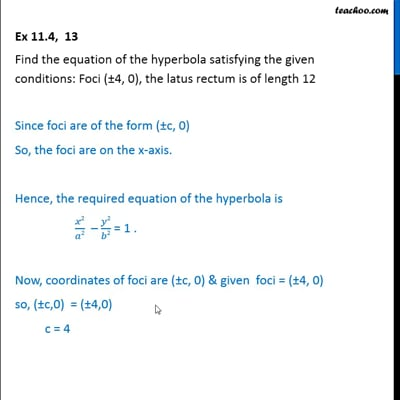This video is only available for Teachoo black users

### Transcript

Ex 11.4, 13 Find the equation of the hyperbola satisfying the given conditions: Foci (±4, 0), the latus rectum is of length 12 Since the foci are on the x-axis. Hence, the required equation of the hyperbola is 𝒙𝟐/𝒂𝟐 – 𝒚𝟐/𝒃𝟐 = 1 Now, coordinates of foci are (±c, 0) & given foci = (±4, 0) so, (±c,0) = (±4,0) c = 4 Now, Latus rectum =2𝑏2/𝑎 Given latus rectum = 12 So, 2𝑏2/𝑎=12 2b2 = 12a b2 = 6a We know that c2 = a2 + b2 Putting value of c & b2 (4)2 = a2 + 6a 16 = a2 + 6a a2 + 6a − 16 = 0 a2 + 8a − 2a −16 = 0 a(a + 8) − 2 (a + 8) = 0 (a − 2) (a + 8) = 0 So, a = 2 or a = -8 Since ‘a’ is distance, it cannot be negative , So a = −8 is not possible ∴ a = 2, From (1) b2 = 6a b2 = 6 ×2 b2 = 12 Thus, Required equation of hyperbola 𝑥2/𝑎2 − 𝑦2/𝑏2=1 Putting values 𝑥2/22 − 𝑦2/12=1 𝒙𝟐/𝟒 − 𝒚𝟐/𝟏𝟐=𝟏